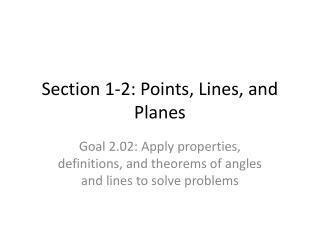DownloadDownload PresentationSection 1-2: Points, Lines, and Planes

# Section 1-2: Points, Lines, and Planes

Download Presentation## Section 1-2: Points, Lines, and Planes

- - - - - - - - - - - - - - - - - - - - - - - - - - - E N D - - - - - - - - - - - - - - - - - - - - - - - - - - -
##### Presentation Transcript

1. Section 1-2: Points, Lines, and Planes Goal 2.02: Apply properties, definitions, and theorems of angles and lines to solve problems

2. Essential Questions • 1.) How do you identify and model points, lines, and planes? • 2.) How are coplanar points, intersecting lines, and planes identified? • 3.) How are the basic postulates and theorems about points, lines, and planes used?

3. space • the set of all points

4. collinear points • points all in one line

5. coplanar points • points all in one plane

6. intersection of two figures • the set of points that are in both figures

7. postulates (or axioms) • statements accepted without proof

8. theorems statements that have been proven

9. 5 Basic Postulates • 1.) A line contains at least two points; a plane contains at least three points not all in one line; space contains at least four points not all in one plane. • 2.) Through any two points there is exactly one line. • 3.) Through any three points there is at least one plane, and through any three noncollinear points there is exactly one plane. • 4.) If two points are in a plane, then the line that contains is in that plane. • 5.) If two planes intersect, then intersection is a line.

10. 3 Basic Theorems • 1.) If two lines intersect, then they intersect in exactly one point. • 2.) Through a line and a point not in the line there is exactly one plane. • 3.) If two lines intersect, then exactly one plane contains the line.

11. Examples, p 13 (1-9 odd) • 1.) A, D, E 3.) B, C, F • 5.) F, B, D 7.) G, F, C • 9.) Name line m in three other ways. • Do p 13 (2-10 even)

12. P 14 (11 - 43 odds) 11.) the bottom 13.) the front 15.) the left side 17.) Planes QRS and RSW 19.) Planes XWV and UVR 21.) QU 23.) XT 25.) R, V, W 27.) U, X, S 29.) T, V, R 31.) plane UVW 33.) plane TUX 35.) point Q with V, W, S 37.) point W with X, V, R 39.) S, U, V, Y 41.) X, S, V, U 43.) S, V, C, Y

13. With partners p 14 (12 – 42 even) • Discuss always, sometimes or never questions • Review counterexample • Together p 15 (60 – 65 all) • Independent Practice: p 16 (85-89 all)

14. Homework • Textbook: p 9 (56 – 59 all) • Workbook: Practice 1-2